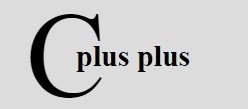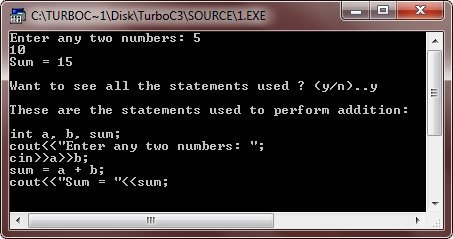# C++ Statements

Statements are the instructions gives to the computer to perform any kind of action, be it data movements, be it making decisions or be it repeating actions. Statements from the smallest executable unit within C++ program.

Statements are terminated with a semicolon (;). The simplest statement is empty, or null statement. It takes the following form :

` ;       // it is a null statement (notice, it is just a semicolon)`

A null statement is useful in those instances where the syntax of the language requires the presence of a statement but where the logic of the program does not.

## Compound Statement - Block Statement

A compound statement in C++ is a sequence of statements enclosed by a pair of braces {}. For example,

```{
statement1 ;
statement2 ;
:
:
:
statementN ;
}```

Represents a compound statement. A compound statement is equivalently called a block. A compound statement or a block is treated as a single unit and may appear anywhere in the program where a single statement may appear. Let's take an example

```/* C++ Statements */

#include<iostream.h>
#include<conio.h>
#include<stdlib.h>
void main()
{
clrscr();
int a, b, sum;
cout<<"Enter any two numbers: ";
cin>>a>>b;
sum = a + b;
cout<<"Sum = "<<sum;
char ch;
cout<<"\n\nWant to see all the statements used ? (y/n)..";
cin>>ch;
cout<<"\n";
if(ch=='y' || ch=='Y')
{
cout<<"These are the statements used to perform addition:\n\n";
cout<<"int a, b, sum;\n";
cout<<"cout<<\"Enter any two numbers: \";\n";
cout<<"cin>>a>>b;\n";
cout<<"sum = a + b;\n";
cout<<"cout<<\"Sum = \"<<sum;\n";
}
else
{
cout<<"Press any key to exit..\n";
getch();
exit(1);
}
getch();
}```

Here is the sample run of the above C++ program:### More Examples

Below are some more C++ programs listed, that you may like:

Tools
Calculator

Quick Links
Signup - Login - Give Online Test